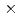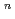This paper describes empirical equation development of crack growth rates (CGR) in consideration of IASCC of neutron irradiated stainless steel to contribute to structural integrity assessment of BWR reactor internals. Empirical equations of CGR (da/dt) were developed based on a formula of da/dt = MK, assuming that "M" and "n" tend to be saturated with increasing neutron fluence. To obtain the empirical equations for normal water chemistry (NWC) and hydrogen water chemistry (HWC) of BWR, a data fitting with least square method was applied to the datasets consisting of F, K and CGR from post irradiation examinations of neutron irradiated stainless steel under simulated NWC and HWC conditions from open literature. As a result, calculated results by the equation for NWC showed good agreement with measured CGR data, meanwhile those for HWC did not. The above difference was seemed to be attributed that CGR data obtained under HWC conditions were scattered extensively.